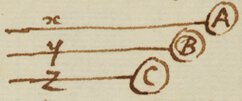<1r>

## Probleme

An equation being given expressing the Relation of two or more lines x, y & z described in the same time by two or more moving bodies A, B, C, & to find the relation of their velocities p, q, r, &c## Solution

Set all ye terms on one side of the Equation that they become equal to nothing. Multiply each term by so many times $\frac{p}{x}$ as x hath dimensions in that term. Secondly multiply each term by so many times $\frac{q}{y}$ as y hath dimensions in it. Thirdly multiply each term by so many times $\frac{r}{z}$ as z hath dimensions in it &c. The summ of all these products shall be equal to nothing. Which Equation gives the relation of p, q, r, &c.

## Or more generally thus.

Order the Equation according to the dimensions of x, & putting a & b for any two numbers whether rational or not, multiply the thers thereof by any part of this Progression $\frac{ap-3bp}{x}.\frac{ap-2bp}{x}.\frac{ap-bp}{x}.\frac{ap}{x}.\frac{ap+bp}{x}.\frac{ap+2bp}{x}.\frac{ap+3bp}{x}.&c.$ Also order the Equation according to the dimensions of y & multiply the terms of it by this Progression $\frac{aq-3bq}{y}.\frac{aq-2bq}{y}.\frac{aq-bq}{y}.\frac{aq}{y}.\frac{aq+bq}{y}.\frac{aq+2bq}{y}.\frac{aq+3bq}{y}.&c$ Also order it according to the dimensions of z & multiply its terms by this Progression $\frac{ar-3br}{z}.\frac{ar-2br}{z}.\frac{ar-br}{z}.\frac{ar}{z}.\frac{ar+br}{z}.\frac{ar+2br}{z}.\frac{ar+3br}{z}$. &c. The summ of these products shall bee equal to nothing. Which equation gives the relation of p, q, r, &c.

## Examples.

Example 1. If the propounded Equation be ${x}^{3}-2xxy+4xx-7xyy-{y}^{3}-103=0$. By the precedent Rule the first operation will produce $3xxp-4xyp+8xp+7yyp$. The second produceth $-2xxq+14xyq-3yyq$. And these two added together make $3xxp-4xyp+8xp+7yyp-2xxq+14xyq-3yyq=0$.

Example 2. If the Equation be ${x}^{3}-2aay+zzx-yyx+zyy-{z}^{3}=0$ the first operation will produce $3pxx+pzz-pyy$, the second $-2aaq-2yxq+2zyq$, the third $+2zxr-yyr-3zzr$. The summ of wch is $3pxx+pzz-pyy-2aaq-2yxq+2zyq+2zxr-yyr-3zzr=0$.

## Demonstration

If two bodies A & B move uniformly, the one successively \succesively/ from a to c, e, g &c the other in the same times from b to d, f, h &c then are the lines ac & bd, ce & df, eg & fh &c in the same proportion to one another as are the velocities p & q wherewith the bodies describe them <2r> And tho they move not uniformly yet are the infinitely little lines wch each moment they describe, as the velocities are which they have while they describe them. As if the body A with the velocity p describe the infinitely little line po in one moment, the body B with the velocity q will describe the line {illeg}qo in the same moment For p.q:::po. {illeg}qo. So that if the described lines be x & y in one moment they will be x+po & y+{illeg}qo in the next moment.

Now if the equation expressing the relation between the lines x & y be for instance $rx+xx-yy=0$